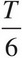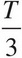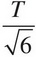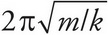# AP Physics 1 Question 249: Answer and Explanation

### Test Information

Question: 249

4. A spring-block simple harmonic oscillator is set up so that the oscillations are vertical. The period of the motion is T. If the spring and block are taken to the surface of the Moon, where the gravitational acceleration is 1/6 of its value here, then the vertical oscillations will have a period of

• A.• B.• C.• D. T

The period of a spring-block simple harmonic oscillator is independent of the value of g. (Recall that T =) Therefore, the period will remain the same.